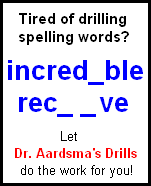Home
Convert 30-Day Trial to Full Registration
Change My Student's Lesson Number
Change the Question Skipping Behavior of My Student's Checker
Choose Problems to be Included in My Students' Lesson
Reset My Student's Current LessonTips for New Users
Use of Calculators in Saxon MathHow to Prepare for ACT/SAT
The Creator of Saxon Math### Change the Skip Mode for Your Student's Checker

Normally the Checker requires the student to complete every problem before allowing the student to advance to the next lesson. This is the Default Mode.

This utility allows the educator to change this default behavior to one of two modes in which the student need only complete every other question before being allowed to advance.

The Even-Only Mode requires the student to do only the even numbered questions in each lesson.

The Even-Even, Odd-Odd Mode requires the student to do only the even numbered questions in even numbered lessons, and only the odd numbered questions in odd numbered lessons.

The student is still required to complete all lesson questions which are labeled alphabetically (i.e., a, b, c...) rather than numerically (i.e., 1, 2a, 2b, 3, ...), and all test questions when using an every-other-question mode.

Once the information requested below has been entered, the educator is sent an e-mail containing a link which may be used to make the change to the selected mode.

NOTE: Use of this utility starts the student back at the beginning of their current lesson. That is, any work already completed by the student on the current lesson will be lost. To avoid loss of work, use this utility only at the start of a new lesson.